TUTORIAL QUESTIONS 145-150

145.  Consider the following standing wave in a medium that is closed at the left end and open at the right end.  The wave velocity in this medium is 4 ft/s, and the medium is 14 ft long.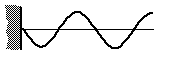Exactly how many seconds after the snapshot above will the wave look as follows: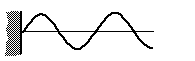Work out the answer and then check it HERE.

Fundamentals of Sound reference: Sec. 2-D.

147.  The following represents a standing wave in a tube of air: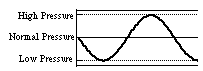a) Both ends are open
b) Both ends are closed
c) Left end is open and right end is closed
d) Left end is closed and right end is open
e) Can't tell anything about the ends

Fundamentals of Sound reference: Sec. 3-A.

148.  The following represents a pressure standing wave in an air tube:Which graph below corresponds to the displacement standing wave?

a)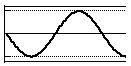b)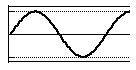c)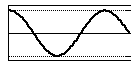d)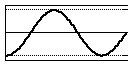Fundamentals of Sound reference: Secs. 3-A, 3-B, 3-D.

150.  Consider the following standing wave in a medium that is closed at the left end and open at the right end.  The wave velocity in this medium is 4 ft/s, and the medium is 14 ft long.What will the wave look like exactly 9.5 seconds after the snapshot above?

a)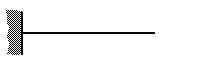b)c)d) None of the above

Fundamentals of Sound reference: Secs. 2-D, 3-D.

Back to Tutorial Listing